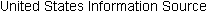# Dimensions of data quality, tuples with missing values, data smoothing and data integration

 Data quality can be assessed in terms of accuracy, completeness and consistency. Propose two other dimensions of data quality. [a] Level of Redundancy: how much of the data is repeated from the various sources one is mining? Redundant data can slow down or confuse the knowledge discovery process. Data reduction and cleaning methods, carefully employed, can aid in removing duplicated data prior to its usage. [b] Level of Normalization: is the data to be analyzed scaled to a specific range such as [0.0, 1.0]? Ex: customer data may contain attributes age and annual_salary. The annual_salary may take more values than age. Hence, if attributes are left un-normalized, then distance measurements taken on annual_salary will most likely outweigh distance measurements taken on age. A solution to this problem is performing data transformation on the data – i.e., normalization and aggregation.In real-world data, tuples with missing values for some attributes are common occurrences. Describe various methods for handling this problem. [a] ignore the tuple(s): done when the class label is missing. Not a very effective method unless the tuple has several attributes w/missing values. [b] fill in the missing value manually: very time consuming; not feasible w/large data sets. [c] use a global constant to fill in missing value: i.e., replace all missing attribute values by the same constant such as the label “missing”. However, the mining program might errantly think a bunch of “missings” are an interesting concept. So, this is not a recommended method. [d] use the attribute mean to fill in the missing value: say you have an average (mean) customer age of 28. This value may be used to fill in all the missing age values. [e] use the attribute mean for all samples belonging to same class as given tuple: Ex: if classifying customers based on credit_risk, replace the missing value w/the know avg_income values for customers in the same credit risk category as that of the given tuple. [f] use the most probable value to fill in the missing value: determined by regression, inference-based tools, or decision-tree reduction. This is arguably the best method for handling missing values. Suppose that the data for analysis include the attribute age. The age values for the data tuples are (in increasing order): 13,15,16,16,19,20,21,22,22,25,25,25,25, 30,33,33,35,35,35,35,36,40,45,46,52,70. [a] Use smoothing by bin means to smooth the above data, using a bin depth of 3. Illustrate your steps. Comment on the effect of this technique for the given data. Each bin value is replace by the mean value of the bin. Bin depth (no. of values in each bin, as per requirements, is 3). (Example of calculation for Bin 1: (13+15+16)/3 = 14.67; other Bins use similar method. Bin 1: 14.67, 14.67, 14.67 Bin 2: 18.33, 18.33, 18.33 Bin 3: 21, 21, 21 Bin 4: 24, 24, 24 Bin 5: 26.67, 26.67, 26.67 Bin 6: 33.67, 33.67, 33.67 Bin 7: 35, 35, 35 Bin 8: 40.33, 40.33, 40.33 Bin 9: 56, 56, 56 [b] How might you determine outliers in the date? Looking at the age values, one might cluster values into “decade groups” such as: The teens cluster (13,15,16,29) The twenties cluster (20,20,21,22,22,25,25,25) The thirties cluster (30,33,33,35,35,35,35,36) The forties cluster (40,45,46) Outliers would be: 52 and 70 [c] What other methods are there for data smoothing? [a] Binning by partition (equidepth of 3). Ex: Bin 1: 13,15,16 Bin 2: 16,19,20 . . . Bin 9: 46,52,70 [b] Binning: smoothening binning boundaries. Find min and max values that define boundaries. Ex: (for equidepth of 3) Bin 1: 13, 13, 16 Bin 2: 16, 16, 20 . . . Bin 9: 46, 46, 70 [c] Use Regression. Linear regression: involves finding the “best” line to fit two variables so that one variable can be used to predict another. Multiple linear regression: extension of linear regression where more than two variables are involved and the data are to fit a multidimensional surface. Regression entails the use of mathematical and statistical techniques. Discuss issues to consider during data integration. Data integration -- “the merging of data from multiple data sources” – is a requirement of data mining. This boils down to combining data from many sources into one “coherent data store”, such as a data warehouse. Issues: [a] Schema integration: A process where the trick is to match up equivalent real-world entities from many data sources. The textbook calls this the “entity identification problem.” Ex.: does product_id in one database and product_number in another refer to the same entity? Often, metadata, found in the DW, can circumvent problems with schema integration. [b] Redundancy: An attribute is redundant IF it can be derived from another table. Ex.: some ones age can be derived from their birth_date. Inconsistencies in attribute or dimension naming can also create redundancies in the data set that results. A mathematical (statistical) scheme, known as correlation analysis, can be used to detect redundancies. Ex.: if you have two attributes A and B, how strongly one attribute implies the other, based on available data, can be determined by this technique. The formula for correlation analysis can be found on pg 113 of the textbook. [c] Relation and resolution of data value conflicts. For a given real-world entity, attribute values may differ depending on source. This can be due to differences in representation, scaling, encoding. Ex.: for a length attribute, a US data source may have measurement values in English (i.e., inches, feet) while a Canadian data source will have the values in metric (centimeters, meters, etc.). top of page More Data warehousing and data mining information: Amazon.com Widgets